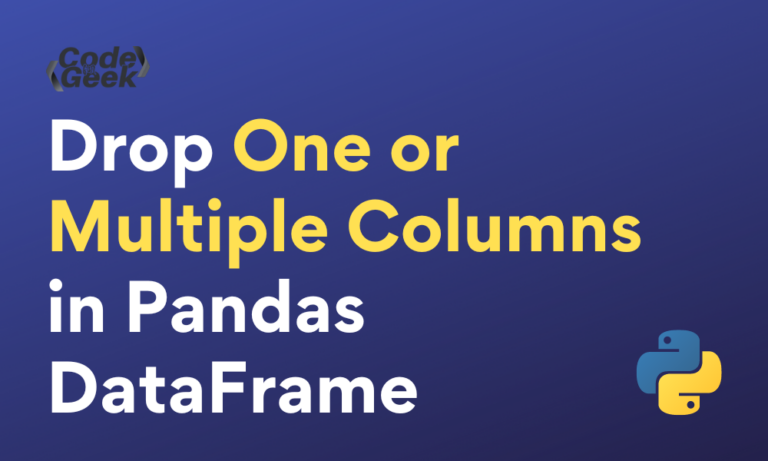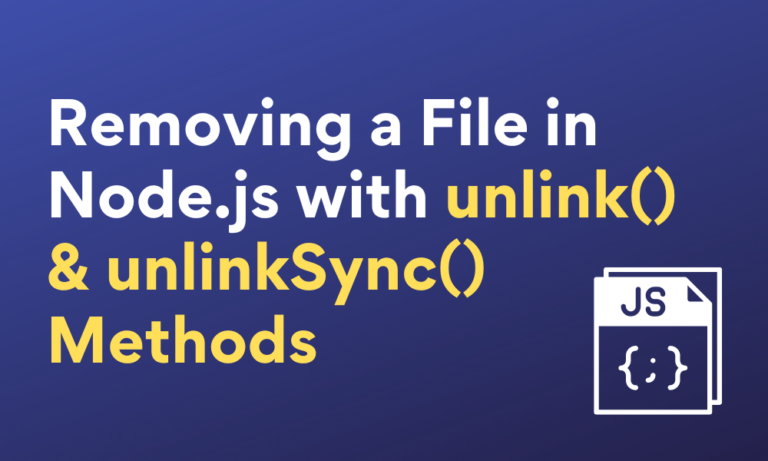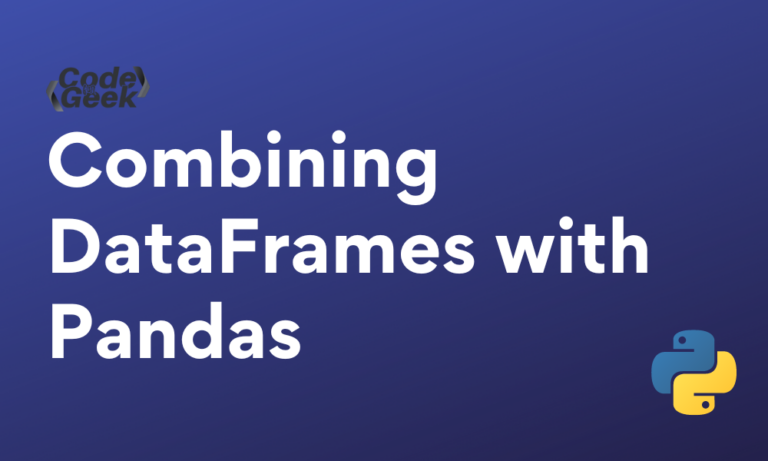# Priyanshu Singh

## How to Drop One or Multiple Columns in Pandas DataFrame (5 Ways)Dropping columns from Pandas DataFrame in Python is a common operation in data analysis and data preprocessing tasks. There are several use cases where we might want to drop specific columns from DataFrame. For instance, whenever we are working with large…In Node.js, the File System module provides various methods for interacting with files. We can use these methods for removing files as well. Here are some use cases where we might need to remove files: There are two ways to…

## Convert Pandas DataFrame To NumPy Array: A Step-by-Step GuideConverting a Pandas DataFrame to a Numpy array is a common operation in various data analysis, machine learning, and scientific computing scenarios. Here are some use cases for converting DataFrame to Numpy array: Converting Pandas DataFrame to NumPy Array in…

## Combining DataFrames with Pandas: Exploring merge(), join(), concat(), and append() MethodsIn this article, we will learn how to combine DataFrames with Pandas in Python. We’ll look at four different methods so that you can choose between them based on your needs. There are several use cases where we might want…